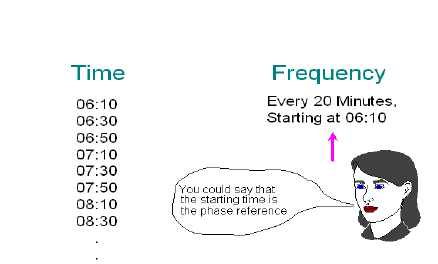# What is Vibration? – Part 2

from DLI Engineering
Posted 11/3/2003

Energy and Power Considerations
Energy is required to produce vibration and in the case of machine vibration, this energy comes from the source of power to the machine. This energy source can be the AC power line, an internal combustion engine, or steam driving a turbine, etc. Energy is defined as force multiplied by the distance over which the force acts, and the SI unit of energy is the Joule. One Joule of energy is equivalent to a force of one Newton acting over a distance of one meter. The physical concept of work is similar to that of energy, and the units used to measure work are the same as those for measuring energy.

The actual amount of energy present in the machine vibration itself is usually not very great compared to the energy required to operate the machine for its intended task.

Power is defined as the rate of doing work, or the rate of energy transfer, and according to the SI, it is measured in Joules per second, or Watts. One horsepower is equivalent to 746 watts. Power is proportional to the square of the vibration amplitude, just as electrical power is proportional to the voltage squared or the current squared.

According to the law of conservation of energy, energy cannot be created or destroyed, but it can be transformed into different forms. The vibratory energy in a mechanical system is ultimately dissipated in the form of heat.

Linear and Non-Linear Systems
To assist in understanding the transmission of vibration through a machine, it is instructive to investigate the concept of linearity and what is meant by linear and non-linear systems. Thus far, we have discussed linear and logarithmic amplitude and frequency scales, but the term “linear” also refers to the characteristics of a system which can have input and output signals. A “system” is any device or structure that can accept an input or stimulus in some form and produce a corresponding output or response. Examples of systems are tape recorders and amplifiers, which operate on electrical signals, and mechanical structures, whose inputs are vibration forces, and whose outputs are vibration displacements, velocities, or accelerations.

Frequency Analysis
To get around the limitations in the analysis of the wave form itself, the common practice is to perform frequency analysis, also called spectrum analysis, on the vibration signal. The time domain graph is called the waveform, and the frequency domain graph is called the spectrum. Spectrum analysis is equivalent to transforming the information in the signal from the time domain into the frequency domain. The following relationships hold between time and frequency:A train schedule shows the equivalence of information in the time and frequency domains:The frequency representation in this case is much shorter than the time representation. This is a “data reduction”.

Note that the information is the same in both domains, but that it is much more compact in the frequency domain. A very long schedule in time has been compressed to two lines in the frequency domain. It is a general rule of the transformation characteristic that events that take place over a long time interval are compressed to specific locations in the frequency domain.

EXPLORE BY TOPIC